# Algebra - math word problems

1. Tractors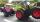Two tractors plow the field in 4 hours together. If the first tractor plow half of the field and then the second tractor completed the job, it would take 9 hours. How many hours does the field plow for each tractor separately?
2. The thirdThe one-third rod is blue, one-half of the rod is red, the rest of the rod is white and measures 8 cm. How long is the whole rod?
3. Sequence 11What is the nth term of this sequence 1,1/2,1/3,1/4,1/5 ?
4. Two pumpsPump A takes twice as long to fill a tank than B . Together they take 2 hours to fill the tank. How long does each one take to fill the tank by itself?
5. Balls groups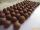Karel pulled the balls out of his pocket and divide it into the groups. He could divide them in four, six or seven, and no ball ever left. How little could be a ball?
6. Four workers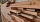Four workers will lay the floor of the gym in six hours. After an hour of working together, one of the workers left to the doctor. How long before the remaining three workers finish their work?
7. The workThe work was to be done by 150 workers. At the beginning of their work, their number reduced by 40, which increased the time of work by 5 and 1/3 of the schedule. How long did work take?
8. Glass panelA rectangular glass panel with dimensions of 72 cm and 96 cm will cut the glazier on the largest square possible. What is the length of the side of each square? How many squares does the glazier cut?
9. TurnersTwo turners perform joint work in 3 hours. The first turner would perform the job himself in 7 hours. How long would the same job last for a second turner if he worked alone?
10. ABCD squareIn the ABCD square, the X point lies on the diagonal AC. The length of the XC is three times the length of the AX segment. Point S is the center of the AB side. The length of the AB side is 1 cm. What is the length of the XS segment?
11. Money 6Lita had some money. She spent 1/2 of it on a hand bag and 1/3 of the remainder of the blouse. She had 260.00. How much money did she have at start?
12. Lcm of three numbersWhat is the Lcm of 120 15 and 5
13. Equilateral triangleA square is inscribed into an equilateral triangle with a side of 10 cm. Calculate the length of the square side.
14. Largest angle of the triangleCalculate the largest angle of the triangle whose sides have the sizes: 2a, 3/2a, 3a
15. Class alphabet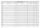All 29 pupils in the class are written in a class by alphabet. The number of pupils enrolled before Paul is three times higher than the number of pupils behind him. Calculate how many pupils are enrolled after Paul.
16. Rectangular trapezoidThe ABCD rectangular trapezoid with the AB and CD bases is divided by the diagonal AC into two equilateral rectangular triangles. The length of the diagonal AC is 62cm. Calculate trapezium area in cm square and calculate how many differs perimeters of the.
17. In and out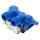The empty tank is filled in 12 minutes and empty in 16 minutes. How long does it take to fill if we forgot to close the drain? If the tank has 1000 l.
18. TogetherGrandfather, father, and son are carpenters. They decided to make a wardrobe. Father produces it for 12 hours, his son for 15 hours, and grandfather for 10 hours. How long will they make it together?
19. Endless lego setThe endless lego set contains only 6, 9, 20 kilograms blocks that can no longer be polished or broken. The workers took them to the gym and immediately started building different buildings. And of course, they wrote down how much the building weighed. They
20. Sick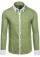Six seamstresses should make 60 shirts in five business days. After three days two seamstresses were sick. How many days have the remaining seamstresses to finish contract?

Do you have an interesting mathematical word problem that you can't solve it? Enter it, and we can try to solve it.

To this e-mail address, we will reply solution; solved examples are also published here. Please enter the e-mail correctly and check whether you don't have a full mailbox.

Please do not submit problems from current active competitions such as Mathematical Olympiad, correspondence seminars etc...